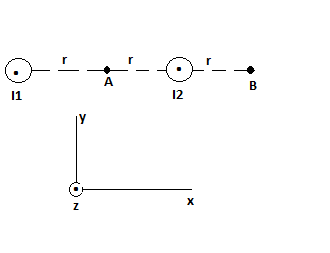# Two long, straight, parallel wires are shown in the figure below. The current in the wire on the...

## Question:

Two long, straight, parallel wires are shown in the figure below. The current in the wire on the left is six times the current in the wire on the right. Find an expression for the magnetic field at points A and B. Use the indicated coordinate system to write your answer in component form. (Use the following as necessary: {eq}I_2, r, \mu_0 {/eq}.)## The Magnetic Field at a point due to a long straight current carrying wire:

The Magnetic Field at a point due to a long straight current carrying wire is given by the equation;

{eq}B = \dfrac{\mu_0 I}{2\pi r} {/eq}

where,

• {eq}r {/eq} is the perpendicular distance of the point from the wire.
• I is the current in the wire and
• {eq}\mu_0 = 4\pi \times 10^{-7} \ \rm Tm/A {/eq} is the permeability of the vacuum.

The direction of the magnetic filed B is the direction of {eq}I\vec{dl} \times \vec{r} {/eq}

where,

• {eq}I\vec {dl} {/eq} is the current element.

Given:

• {eq}I_1 = 6I_2 {/eq}

The diagram showing the magnetic fields at point A and B due to parallel current carrying wires as shown in the figure 1 ...

Become a Study.com member to unlock this answer! Create your account